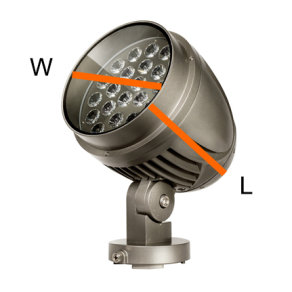# Effective Projected Area RatingsExterior installations can involve multiple aspects of examination that are not involved with interior installations, including environmental conditions such as rain, snow, sleet, ice, water, sunlight, and wind. For this study, we will focus on the impact of wind on lighting fixtures. Designing an exterior installation, the consideration of wind isn’t as simple as selecting a low-profile fixture. One must consider multiple other aspect, such as the wind speed rating for the location of the install (please see the Wind Map in the American Society of Civil Engineers (ASCE) 7-10 International Building Code manual), the height of the install, the size (area) of the fixture(s), the weight of the fixture(s), the degree of tilt of the fixture(s), the listed EPA rating of any bracket or accessory attached to the fixture(s) or the fixture(s) will be mounted to, and more. Pole and bracket manufactures will publish the EPA ratings and limitations of their product. Fixture manufacturers will publish the EPA ratings of their product or have the data available to send when inquired about.

What is EPA?
EPA is the Effective Projected Area (wind load) of an object and/or structure. EPA is calculated for the worst-case scenario of exterior installations.

Calculating EPA
Calculating the EPA or the wind load is done using the following formula: F = A x P x Cd.

In this formula, F (force or wind load) equals A (projected area of the object) multiplied by P (wind pressure) multiplied by Cd (drag coefficient). This formula is not for planning new construction but rather a basic formula for existing retrofits.

New construction projects require the Electronic Industries Alliance Formula: F = A x P x Cd x Kz x Gh.

In this formula, F (force) = A (projected area) multiplied by P (wind pressure) multiplied by Cd (drag coefficient) multiplied by Kz (exposure coefficient) multiplied by Gh (gust response factor).

Projected area (A) is found by calculating the three-dimensional shape of the fixture or object, which is dependent upon the shape and size of the fixture or object. A standard box-shape or flat-shape would involve Length x Width. A circular/round-shape would be Length x Width, where the width is the diameter of the circular/round-shape (See image below).

Wind Pressure (P) is found by calculating the wind speed (v) squared multiplied by 0.00256. In short, P = v² x 0.00256. The unit for P will always be listed as pounds per square foot (psf).

The standard Drag Coefficient (Cd) of a short circular/round shape is 0.8, 1.2 for a long circular/round-shape, and 1.4 for a box/flat-shape fixture or object.

The Exposure Coefficient (Kz) is found by calculating the height from the ground to the midpoint of the fixture/object (z) divided by 33(²/7). In short, Kz = [z/33](2/7).

Finally, the Gust Response Factor (Gh) is calculated by entering the height (h) of the fixture/object into the following formula: Gh = 0.65 + 0.60/[(h/33)(1/7)].

The structure manufacturer will often publish their product based on wind speed mile per hour (MPH) on a 1.3 gust factor. The maximum EPA (SQFT), along with the maximum weight (LBS) allowed for that structure will be provided in the specification data. Refer to the Wind Map to help determine the best structure to use for your install, then limit the number of fixtures and brackets/accessories you use on that structure so as not to exceed the maximum EPA and maximum weight.

Lets put this knowledge to use:
For example, a 25ft round tapered steel pole with a 100 MPH w/1.3 Gust would have a maximum EPA of 8.0 to 19.8 SQFT [dependent upon the wall thickness] and maximum weight allowance of 200-495 LBS. A flood/washer fixture chosen for the install might have an EPA of 2.2 SQFT and a weight of 46 LBS. If the 25ft round tapered steel pole chosen has a maximum EPA of 8.0 SQFT and maximum weight of 200 LBS, then you would be limited to 3 of the selected flood/washer fixtures per pole because the EPA of the collective 3 units would be 6.6 SQFT and the weight would be 138 LBS. In this scenario, you still have some weight tolerance remaining, but adding another fixture would exceed the 8.0 SQFT EPA tolerance.

Aside from EPA, there are several other factors that come into play, depending on the structure of the install, including impact rating (IK Rating) and vibration rating (IE: 3G, 4G), which may be discussed in later entries A safe installation will account for all these variables.

With the EPA in hand, you can start building a safe installation.